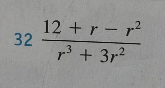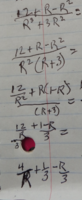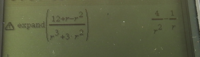# this stumps me:

#### allegansveritatem

##### Full Member
I came across this problem at the end of one section of the algebra review in my precalculus. I will post the problem and how I tried to work it out--it has been asked of us to simplify the expression:
Problem:Here is how I tried to simplify this:Here is what I got from my calculator:I really have no idea how the calculator got this and I really have no idea how to simplify this expression. I tried to divide each component of the numerator by the denominator. I mean, what else can you do when neither the numerator or denominator can be fully factored.

#### topsquark

##### Full Member
Hint: Factor the numerator of the original expression...

-Dan

•MarkFL

#### Otis

##### Senior Member
Can you factor -(r^2 - r - 12) ?•Jomo

#### pka

##### Elite Member
$$\displaystyle \dfrac{12+r-r^2}{r^3+3r^2}\\\dfrac{(4-r)(3+r)}{r^2(r+3)}\\\dfrac{4-r}{r^2}$$

#### allegansveritatem

##### Full Member
$$\displaystyle \dfrac{12+r-r^2}{r^3+3r^2}\\\dfrac{(4-r)(3+r)}{r^2(r+3)}\\\dfrac{4-r}{r^2}$$
Oh boy! I misread the thing...somehow I missed the fact that the numerator is a quadratic expression. What a dope! Thanks for pointing this out. Sometimes it happens...the answer is right there hanging out for all the world to see and...I don't see it. At least I did factor the denominator.

#### allegansveritatem

##### Full Member
Can you factor -(r^2 - r - 12) ?I see it now but somehow the fact that this is a quadratic expression was completely lost on me. No way to excuse this...I have seen plenty like this with constant out front, but somehow I missed this one. Thanks for replying

#### allegansveritatem

##### Full Member
Hint: Factor the numerator of the original expression...

-Dan
Right. I see it now. Thanks

#### Otis

##### Senior MemberAnytime you're given a rational function (i.e., algebraic fraction), you should first consider factoring everything. That effort is not always needed, but you never know when some exercise might be cooked up for simplification(s).

Cheers#### topsquark

##### Full Member
I should mention, though it is not always required for full marks, that $$\displaystyle \dfrac{4 - r}{r^2}$$ is not quite the correct answer. Since we canceled the r + 3 we need to specify that $$\displaystyle r \neq -3$$ for our expression since that is not allowed in the original expression . So the correct answer is actually
$$\displaystyle \dfrac{4 - r}{r^2} \text{ such that } r \neq -3$$

Always look for something like this.

-Dan

•Otis

#### ksdhart2

##### Senior Member
I went at this again today and here is what I got. It is not quite what the other posters have...but I think that is attributable to some kind of synonomy?

Or have I made some kind of mistake? I have 1/R instead of -R/R2 because -R divided by R2 is -1. No?

$$\displaystyle \frac{{\color{red} \textbf{-} } R^2 + R + 12}{R^3 + 3R^2}$$

But in the next step, the negative sign has disappeared:

$$\displaystyle \frac{(R + 4)(R + 3)}{R^2(R + 3)}$$

If you re-multiply this out, you'll get $$\displaystyle {\color{red} \textbf{+} }R^2 \cdots$$ which should set some alarms blaring.

#### pka

##### Elite Member
I went at this again today and here is what I got. It is not quite what the other posters have...but I think that is attributable to some kind of synonomy?

Or have I made some kind of mistake? I have 1/R instead of -R/R2 because -R divided by R2 is -1. No?
$$\displaystyle \dfrac{4-r}{r^2}=\dfrac{4}{r^2}-\dfrac{1}{r} \: (r\ne 0)$$

#### Jomo

##### Elite Member
In my opinion, the final answer should be a single fraction. That is although (4/r2) - (1/r) does equal (4-r)/r2, I feel that the final answer should be (4-r)/r2 where r$$\displaystyle \neq$$0

#### pka

##### Elite Member
In my opinion, the final answer should be a single fraction. That is although (4/r2) - (1/r) does equal (4-r)/r2, I feel that the final answer should be (4-r)/r2 where r$$\displaystyle \neq$$0
Why do you think that? If this is an online class or an online test then the correct answer is the one expected by the machine and not the one you may prefer.

#### allegansveritatem

##### Full Member

$$\displaystyle \frac{{\color{red} \textbf{-} } R^2 + R + 12}{R^3 + 3R^2}$$

But in the next step, the negative sign has disappeared:

$$\displaystyle \frac{(R + 4)(R + 3)}{R^2(R + 3)}$$

If you re-multiply this out, you'll get $$\displaystyle {\color{red} \textbf{+} }R^2 \cdots$$ which should set some alarms blaring.
I don't see what you mean here. I do have a negative sign--although I admit it is not very easy to make out--with (-r+4).

#### Jomo

##### Elite Member
Why do you think that? If this is an online class or an online test then the correct answer is the one expected by the machine and not the one you may prefer.
Well first of all we were not given choices. It looks neater when a solution is in factored form as a single fraction. I am sure that you would not expand a denominator if it was in factored form. I guess though that it is a matter of style (if the solutions are not given.

#### ksdhart2

##### Senior Member
I don't see what you mean here. I do have a negative sign--although I admit it is not very easy to make out--with (-r+4).
Oh, yes. I see it now that I know it's there. I genuinely thought it was part of the R.

#### Otis

##### Senior Member
… -R divided by R2 is -1
That is a typo, yes?

The answer shown in your work is correct (post #10). So is topquark's answer (post #9). These two answer forms are equivalent. As a precalc student, you need to recognize the following.

$$\displaystyle \frac{A - B}{C} = \frac{A}{C} - \frac{B}{C}$$

PS: Don't forget to specify that R ≠ -3 (Your instructor will take note and be pleased!)

Cheers•topsquark

#### Denis

##### Senior Member
I came across this problem at the end of one section of the algebra review in my precalculus. I will post the problem and how I tried to work it out--it has been asked of us to simplify the expression:
Problem:View attachment 11369
Easier if you multiply by -1 right away:
(r^2 - r - 12) / -(r^3 + 3r^2)

Now factor numerator...